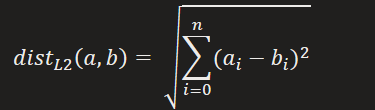TowardsMachineLearning# Introduction

Word2Vec was developed at Google by Tomas Mikolov, et al. and It uses Neural Network with one hidden layer to learn word embeddings.

The beauty with word2vec is that the vectors are learned by understanding the context in which words appear. The result are vectors in which words with similar meaning end up with a similar numerical representation.

## Why Word2vec is so Important / Why Word2vec got so popular?:-

To understand this let’s first understand how word2vec is different than any other Vector Space Model.

Suppose, you have a list of documents associated to a word (where the word appear), and if you use
concepts like DTM / TF-IDF, that word will be associated with the TF / TF-IDF values of its document list.  These values / scores tell us about the occurrence or importance of word in a document but it doesn’t really talk about any semantic or syntactic relationship between two words.

On the other hand, more advanced vector representations of terms, such as word2vec or other distributed or distributional representation, are based on the distributional hypothesis (Harris, 1954): you can determine the meaning of a word by looking at its company (its context). If two words occur in a same “position” in two
sentences, they are very much related either in semantics or syntactics.

Weights of vectors are learnt (unsupervised) using neural network architecture (not really a neural network though), and scores/values in concepts like DTM or tf-idf are solely counted, not learnt. This is a big deal and greatly improve machine learning models that utilize text as input.

## What are Word vectors /Word Embeddings Anyway?:-

Word vectors, also called word embeddings, represent words in a way that:

1. encodes their meaning and
2. allows us to calculate a similarity score for any pair of words.

The similarity score is simply a fractional value between -1.0 and 1.0, with higher values corresponding to higher
similarity.

This Google News pretrained model contains vectors for 3 million words (including multi-word names or concepts like “Elon_Musk” or “computer_science”). If I take their vector representing the word “couch”, and their vector representing the word “book”, I can do some math (which I’ll get to in a minute) to compare their vectors. This gives a score of 0.12–not very similar. If I do the same thing with “couch” and “sofa”, I get a score of 0.83, reflecting the fact that these words are synonyms.

Being able to compare two words in this way also means that you can compare a word to an entire vocabulary of words, and identify the most similar words in that vocabulary. For example, the three most similar words to “couch” in Google’s model are “sofa”, “recliner”, and “couches”.

## Feature Vectors & Similarity Scores

Word vectors represent words in a way that encodes their meaning. A vector is simply an array of fractions. Here’s a vector taken from the Google News model. It’s the vector for the word “fast”. It consists of 300 floating point values (I’ve only displayed a handful of them): <0.0575, -0.0049, 0.0474, …, -0.0439>

you should think of a word vector as a point in high-dimensional space.

The straight line distance between two points is called the “Euclidean” or “L2” distance, and itcan be extended to any number of dimensions. Here’s the formula for the distance between two vectors a and b with an arbitrary number of dimensions (n dimensions):Here’s the important insight, word2vec learns a vector for each word in a vocabulary such that words
with similar meanings are close together and words with different meanings are farther apart. That is, the Euclidean distance between a pair of word vectors becomes a measure of how dissimilar they are.

But wait, didn’t we talk earlier about word vector comparisons in terms of a similarity score, from -1.0 to 1.0? The Euclidean distance is what we intuitively understand as distance, and it’s a workable distance metric for comparing word vectors, but in practice another metric called the Cosine similarity gives better results.

\displaystyle Similarity(A,B)=\frac{{A.B}}{{||A||*||B||}}=\frac{{\sum\nolimits_{{i=1}}^{n}{{{{A}_{i}}*{{B}_{i}}}}}}{{\sum\nolimits_{{i=1}}^{n}{{{{A}^{2}}_{i}}}*\sum\nolimits_{{i=1}}^{n}{{{{B}^{2}}_{i}}}}}

The Cosine similarity between two vectors a and b is found by calculating their dot product, and dividing this by their magnitudes. The cosine similarity is always a value between -1.0 and 1.0.

To be continued…..

In next post we’ll take deep dive in inner working of word2vec algorithm. So stay tuned!

## Article Credit:-

Name:  Praveen Kumar Anwla
Founder:  TowardsMachineLearning .Org